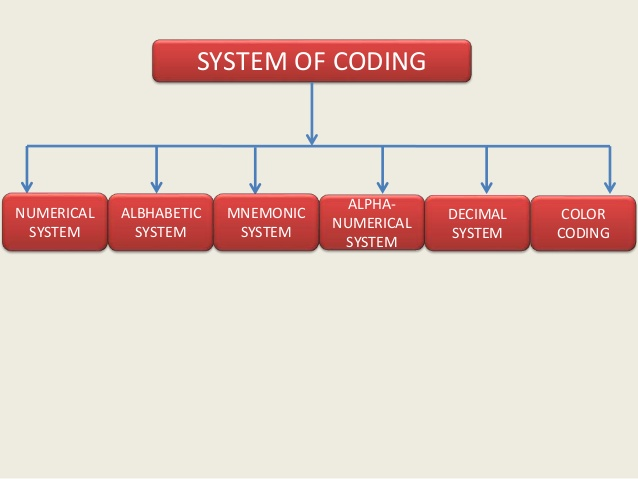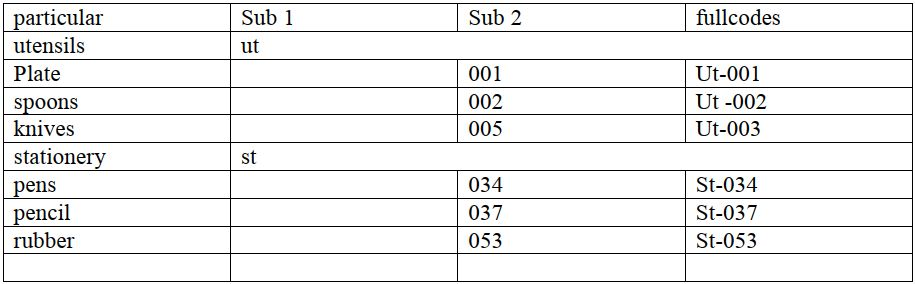# Methods and bases of coding materials

There are various ways used in coding materials however the principle system of coding commodities used are

1. Alphabetic coding system
2. Numeric coding system
3. Alphanumeric coding system### Numeric coding system

As the name suggests numeric system involves the use of numbers whenever one is allocating codes to materials

Numeric system is sub divide

1. Simple numbers
2. Block numbers
3. Stroke/dash
4. Decimals

Simple number

Each item is located a number and a few numbers are left out to give room for expansion

Block number

Goods are classified into groups and given a block number Example raw materials can be given (1-500) as its block numbers. Dash /stoke

This is an improvement of the block numbers system.in this system a dash or a stroke is added to describe the various materials in the warehouse.

Decimal numbers

This broadly based on dash or stroke numerical coding system
This system envisage the division of the code and item of stores department into three categories

1. The first category is the division of the materials into main classification eg raw materials. Finished materials package etc.
2. Further divides the main classification into different sub classification according to nature, use quality characteristics
3. Further divides the sub division of materials into different marketability, how frequent its used etc.

Under decimal system each of the categories explained is indicated and separated by a decimal
.the digits may be as many as required but generally should not exceed four.

### Alphabetic coding system

Under this system the materials are allocated a unique alphabet.The first alphabet of a material may be used at the start followed by their precise character of the material Eg utensils –UT

The coding system may how ever be limited to small warehouses.

### Alphanumerical system

As the name implies this coding system involves the combining of both alphabets and numbers.this system was developed in order to take advantage of both the numeric and alphabetic systems. To work out this codes the materials are first grouped them special codes are then given to them. N/B: Under this system, the alphabets always come first### Advantages / Importance of Codification

1. To identify correctly
2.  To avoid long and unwieldy description.
3. To have accurate and logical identification.
4. To prevent duplication.
5. To standardize items.
6. To reduce varieties.
7. To have an efficient purchasing department.
8. To obtain efficiency in recording and accounting.
9. To simplify and facilitate mechanical recording.
10. To simplify and facilitate pricing.
11. To have proper system of location and indexing.
12. To assure correct and efficient inspection; and
13. To implement production as planned